# Diagnosis of Leaf Surface Disease Using Two Datasets of Tomato and Rice Obtained from Image Processing Techniques

Diagnosis of Leaf Surface Disease Using Two Datasets of Tomato and Rice Obtained from Image Processing Techniques

MA of Department of Agriculture Engineering, Payame Noor University (PNU), Mashhad 6481665475, Iran

MA of Department of Agriculture Engineering, Payame Noor University (PNU), Tehran 6461815500, Iran

MA of Department of Agriculture Engineering, Payame Noor University (PNU), Shira 6461891936, Iran

Corresponding Author Email:
m_hosseini@jsu.ac.ir
Page:
77-85
|
DOI:
https://doi.org/10.18280/rces.080303
8 August 2021
|
Accepted:
12 Spetember 2021
|
Published:
30 September 2021
| Citation

OPEN ACCESS

Abstract:

It is of a great importance in modern agriculture to study fast, automatic, inexpensive and accurate method of diagnosing plant diseasesTherefore, timely and accurately diagnosis of the disease in the fields is one of the most important factors in dealing with plant diseases. For this reason, in the present study, the image processing method study, has been examined for diagnosing the two important diseases of rice and tomato, brown spots and leaf blasts. In this study, firstly the data section is treated using improved k-means segmentation, after preprocessing. Secondly, comprehensive features are extracted and the disease areas are demarcated. An improved genetic algorithm is used in the feature selection step. Finally, images are categorized using the k-nearest neighbor’s algorithm (k-NN) classifier. The accuracy of the proposed method for the rice data set is 99.12 and for the tomato data set is 97.29, which shows a very good performance compared to other methods.

Keywords:

modern agriculture, means segmentation, comprehensive features, k-nearest neighbor’s algorithm

1. Introduction

Plants and fruits disease, have caused a significant reduction in the quality and quantity of agricultural products. Farmers usually suffer from growing problems related with variety of plant and fruit diseases. Sometimes herbalists are unable to diagnose the right type of diseases, which can lead to the loss of the product if the plant is not cared for and treated at the right time .

Currently, plant pathologists are pursuing a tedious approach that generally relies on predictions with naked eyes to classify plant disease. The manual methods are not only a time-consuming procedure, but they also lead in to inaccurate results. To minimize product losses, early diagnosis of the disease is needed for preventive action .

Automatic diagnosis of plant and fruit diseases is an important research topic in monitoring agricultural products and automatic diagnosis of the signs and symptoms of the disease rapidly appearing on plant leaves . In addition, since millions of liters of toxic solution are used annually to control pests, weeds and plant diseases, it is necessary to take needed actions in order to better use of these substances and reduce their consumption, which are harmful to the environment . Now days, image processing applications are growing . Many researches in medicine, robotics, meteorology and agriculture, have been done and its applications in the named fields are becoming prevalent. The application of image processing techniques in agriculture is expanding exponentially from farm management to disease diagnosis .

Therefore, image processing is a non-destructive method that uses existing equipment such as cameras, computers, scanners and image analysis programs to collect, record images, process images and analyze the information extracted from the images. Investigating the possibility of using image processing technology in agriculture in various fields such as monitoring the optimal growth of crops, estimating the time of crop production, weeding machines and pesticides has been a topic of interest in recent years. Studies show that image processing techniques can be successfully used in diagnosing the disease .

The purpose of this paper is to investigate the capability of the image processing techniques in the diagnosis of plant disease. After pre-processing, the disease area is divided according to the improved K-means algorithm. Then, the textureal and shape properties are extracted using the Local binary patterns (LBP) and Harlick Gray Level Co-occurrence Matrix1 (HGLCM) binary pattern and closed boundary areas by the maximally stable extremal regions (MSER) algorithm . Then, the improved genetic method  is used to find the optimal features. Finally, the KNN algorithm is used to classify and diagnose plant disease.

The structure of the rest of the article will be as following: The second part is an overview of known methods in the field of plant disease. In the third section, the proposed method for diagnosing plant species will be presented. The fourth section discusses the evaluation of the studied methods along with the results table, their comparisons, analysis and the general conclusion is expressed in the fifth section.

2. Related Work

A method based on image processing techniques and pattern identifying methods to diagnose apple leaf disease was presented in a way that, in the preprocessing stage, first the color change structure was designed for RGB input (red, green and blue) and then the RGB model was converted to HSI (color, saturation and intensity), YUV and gray models . In the segmentation stage, the background is removed based on a certain threshold value and then the image was segmented by the disease point with the region growing algorithm. In feature extraction stage, 38 texture, shape and color features was extracted, which a combination of genetic algorithm and feature selection based on correlation to reduce their dimensions were applied. The classification accuracy for this method has been evaluated to be 0.90.

Sunflower leaf disease was diagnosed and classified. In this method, after preprocessing operation, the leaf segmentation was done using the Particle swarm optimization (PSO) algorithm in order to optimize the clustering process . The average accuracy considered for the classification was 0.98. The reduction of speed and the need for the faster segmentation algorithm were the major problems related to this method.

In another study  Artificial bee colony (ABC) has been used to identify and classify diseases in grape leaves using the colony characteristics of artificial bees. After pre-processing and removing the noise of the leaves, the characteristics of texture, shape and color were extracted. Finally, the features reduced by ABC are given to SVM in the classification stage. The accuracy of the proposed method was predicted to be 0.93. Lack of proper segmentation process was found to be one of the problems of this method.

In another study, rice plant diseases were diagnosed and classified using convolutional neural network. In this method, a large-scale architecture such as VGG16 and Incep-tionV3 was set up to identify diseases and pests. There was also a small two-step architecture for working with mobile devices. The accuracy of the proposed method was predicted to be 0.93.

Rice disease was specifically addressed. Pictures of disease symptoms on leaves and stems are taken from rice fields . A total of 120 images of sick rice plants have been collected from real farm conditions, according to the decision tree classification. The classification accuracy for the decision tree was 0.57 and Random Forest 0.76.

The improved method of randomized forest classification provided a multi-class method for the problem of rice disease classification . This method was a combination of random forest machine learning algorithm and feature evaluation method and a sample filter. This approach aimed to improve the performance of the Random Forest algorithm with the accuracy of 0.97.

Another method  was to classify five types of tomato diseases that was done using the characteristics of shape, texture and color. The classification accuracy for this method was 0.97.

The study  aimed to address the issue of fake positive rates and class imbalances by implementing a filtering framework for plant tomato diseases and pest control. This was done using the CNN classifier. The classification accuracy for this algorithm was 96%.

In addition, the authors  proposed a method for detecting tomato leaves using the VGG16 and transfer learning architecture, which has been found to have 89% accuracy.

3. Dataset

To test the proposed method, Rice disease dataset which can be downloaded from UCI (https://archive.ics.uci.edu/ml/datasets/Rice+Leaf+Diseases). Figure 1 has been used . This dataset contains 120 images of three classes/diseases including Bacterial leaf blight, Brown spot, and Leaf smut, each having 40 images. Also, Tomato disease dataset consists of 6 diseases and a healthy which can be downloaded from https://www.kaggle.com/emmarex/plantdisease (Figure 1, 2) .

## 1.png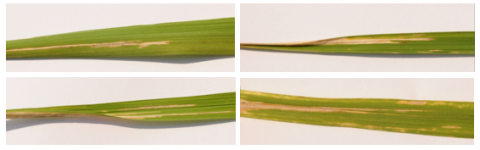Figure 1. Rice disease dataset leaf images

## 2.png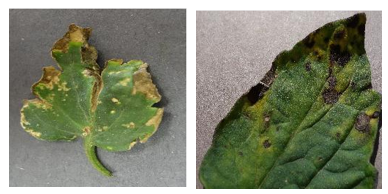Figure 2. Tomato disease dataset leaf images

4. Methodology

In the proposed method, after pre-processing, improved k-means segmentation is performed. In the feature extraction stage, complete features such as geometric, textural and demarcation features are extracted from the images. Then, to avoid feature saturation, an optimized genetic algorithm will be used to select the optimal features. Finally, the KNN classifier classifies the features.

4.1 Pre-processing

In the preprocessing step, the color space of RGB (Red Green blue) is converted to HIS (Hue, Saturation Intensity) space. The goal of achieving color space is to find an algorithm that amplifies disease symptoms in high-resolution images. The ultimate accuracy of the algorithm in diagnosing and classifying the disease largely depends on the selected color space. In this method, in addition to RGB color space, HSI color components were also examined to determine the best color component that can highlight the diseased parts in the best way. Components H, S, and I indicate color difference, saturation, and color, respectively.

4.2 Improved K-means segmentation

This method, despite simplicity, is a basic method for many other clustering methods (such as fuzzy clustering). This method is an exclusive and flat method. Different styles and methods have been expressed for this algorithm.

In this section, the improved k-means algorithm is used for segmentation. This method is known as Kmeans segmentation with Neutrosophic logic used in the study . The clustering process is described as follows:

• At this point, a pixel called P is selected with the least unknown value so that it is the closest to the local average, which has not been assigned to any cluster so far.
• In this step, the same cluster is assigned to a pixel (P) and to its neighbor (N) whose correct difference is less than or equal to the predefined threshold value or the same as | T (P) - T (N) | ≤ Th.
• Steps one and two are repeated until each pixel is assigned to the C cluster.

The pseudo-code for Neutrosophic Cluster Formation is shown in Figure 3.

## 3.png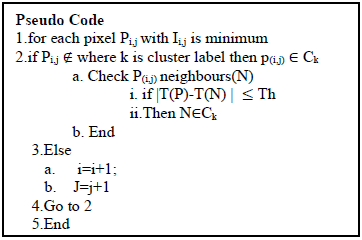Figure 3. Pseudo-code for cluster filtration 

After the refining step, each pair of clusters are merged into a single cluster based on the difference in size and correct values. This makes smaller clusters to be merged into larger clusters, but not vice versa. The pseudo-analysis code of this clustering is shown in Figure 4.

## 4.png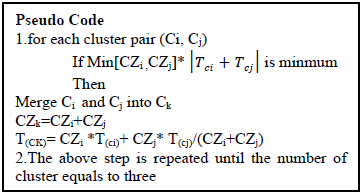Figure 4. Pseudo code for segmentation 

4.3 Extract features

4.3.1 Geometric features

Geometric features extracted from the image after improved K-means segmentation include the following. It should be noted that in all relations M and N, the dimensions of the image, x and y are also related to the coordinates of each pixel and also I, is the segmented image .

Area $=\sum_{x=1}^{M} \sum_{y=1}^{N} \mathrm{I}_{x, y}$        (1)

Perimeter $=2 \times($ length $+$ width $)$      (2)

Majoraxis $=\mathrm{x}+\mathrm{y}$        (3)

In above equation axis = (x, 0) − (−x, 0) and d length of major axis = 2x.

Minoraxislength $=\left((x+y)^{2}+F^{2}\right)^{\frac{1}{2}}$     (4)

Semiaxislength $=c=\sqrt{x-y}$       (5)

Circularity $=2 \times \frac{22}{7}\left(\frac{\sum_{x=1}^{\mathrm{M}} \sum_{y=1}^{\mathrm{N}} \mathrm{I}_{\mathrm{x}, \mathrm{y}}}{(\text { length }+\text { width })^{2}}\right)$                  (6)

Solidity $=\frac{\text { Area }}{\xi}$         (7)

In Eq. (8), ξ denotes the convex area.

mean $=\sum_{(x, y)=1}^{M N} I_{x, y}$     (8)

harmonic mean $=\frac{n}{\sum_{i=1}^{M N}\left(\frac{1}{X_{i}}\right)}$       (9)

Smooth $=1-\frac{1}{1+\sum_{\mathrm{x}, \mathrm{y}} \mathrm{I}_{\mathrm{x}, \mathrm{y}}}$     (10)

Energy $=\sum_{(x, y)=1}^{M N}\left(E_{x, y}\right)^{2}, E_{x, y}=\frac{P_{x, y}}{M N}$        (11)

Px,y which is an element of the GLCM matrix is also gray level co-occurrence matrices (GLCM).

Kurtosis $=\sum_{i=1}^{M N} \frac{\frac{\left(X_{i}-\hat{X}\right)}{M N}}{\sigma^{4}}$           (12)

$\mathrm{Xi} \in(\mathrm{I}(\mathrm{x}, \mathrm{y}))$ and $\hat{X}$ is $\mathrm{I}(\mathrm{x}, \mathrm{y})$ mean.        (13)

$\sigma=\sqrt{\frac{1}{M N}\left(\frac{\left(X_{i}-\hat{X}\right)^{2}}{M N}\right)}$       (14)

Full names of authors are required. The middle name can be abbreviated.

4.3.2 Harlick GLCM (HGLCM) features

In This section, after segmentation, the method of extracting of texture-based statistical features is applied in the proposed method. HGLCM properties are calculated from a statistical distribution which is a combination of the appropriate intensity of each image at specified positions. The classification of images depends on the intensity of the pixels and these statistics. HGLCM is typically used to analyze the second-order probability status of an image, of which 14 features are used in the proposed method .

4.3.3 Maximally stable extremal regions feature (MSER)

The MSER descriptor being applied for extracting point properties, is used in the proposed method to distinguish boundary areas from post-segmentation images .

In MSER, the threshold is considered as relation (15):

$f_{\theta}(x, y)=f(z)=\left\{\begin{array}{c}1, I_{z} \geq 0 \\ 0 \text { otherwise }\end{array}\right.$       (15)

Thus f (z) ∈ (x, y) defines the value of the threshold, which represents values greater than the white threshold and smaller than the black threshold.

Distinguishing of the local extrema edges from the gray-level highly contrasted images; the mapping of the upper layers set would be determined as:

$\chi_{\lambda}(V)=\left\{u \in \mathrm{R}^{2}, \mathrm{v}(u) \geq \lambda\right.$        (16)

$V(\mathrm{u})=\sup \lambda \in \mathrm{R}, \mathrm{u} \in \chi_{\lambda}(V)$        (17)

This is while in above relationships V (u) = R2⟶R at λ level. extracts spots and local features in the image. Eq. (18) is defined for the stability of these properties.

$\varphi=q(i+b)-q(i-d) / q(i)$      (18)

Φ is also defined as follows:

$\varphi=R_{i}^{a}=\frac{\left(\left|R_{i}^{a-\Delta}\right|-\left|R_{i}^{a+\Delta}\right|\right)}{R_{i}^{a}}$       (19)

In the above relation, $R_{i}^{a}$ a represents the interconnected region and R_i represents the local minimum. $R_{i}^{a-\Delta}$ and $R_{i}^{a+\Delta}$ are the local area up and down, respectively. ∆ and R are the extremum regions used to control the MSER parameters. Moreover, change in the amount of intensity in different areas is defined by Eq. (20):

$\frac{|R(+\Delta)-R|}{|R|}$    (20)

Finally, the property vector obtained from this part is N * 64.

$\chi_{\lambda}(V)=\left\{u \in \mathrm{R}^{2}, \mathrm{v}(u) \geq \lambda\right.$    (21)

4.3.4 LBP features

The original LBP operator has been introduced as a powerful descriptor for image texture. This operator generates a binary number for each pixel according to the 3*3 labels of vicinity pixel. Labels are obtained from thresholding the amount of neighboring pixels with the center pixel value in a way that, for pixels with a value greater than or equal to the value of the center pixel, label 1 is placed, and for pixels with values smaller than the value of the center pixel, label 0 is placed. then these labels are placed next to each other in rotation to form an 8-bit number as can be seen in Figure 5.

## 5.png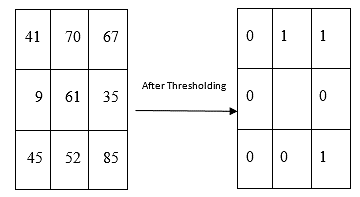Figure 5. LBP method

4.3.5 Gabor features

The methods presented so far differ from each other in how the features are extracted. They also vary in the type of classification system used, and none of the methods has performed a complete classification. Finally, the use of combined orientation methods can increase accuracy. Therefore, the use of a combination of texture Gabor features can be efficient .

The Gabor filter used in the proposed method is created according to the size of the image. Eq. (22) describes how to calculate the Gabor filter.

$G(\mathrm{x}, \mathrm{y})=\frac{\mathrm{f}^{2}}{\pi \gamma \mu} \exp \left(-\frac{\mathrm{x}^{\prime 2}+\mathrm{y}^{\prime 2}}{2 \delta^{2}}\right) \exp \left(\mathrm{j} 2 \pi \mathrm{fx}^{\prime}+\varphi\right)$

$\mathrm{x}^{\prime}=x \cos \theta+y \sin \theta$

$\mathrm{y}^{\prime}=-x \sin \theta+y \cos \theta$                 (22)

In Eq. (20), the Gabor filter is normalized in the frequency domain, which in the above equations f is the sine factor frequency. θ also shows the direction of the normal corrugated lines of the Gabor function to the parallel corrugated lines of the Gabor function. Φ is the phase offset, and δ is equal to the Gaussian cap standard deviation. γ is the spatial visibility ratio that determines the elliptical support of the Gabor function .

4.4 Features select

Feature selection is a process that improves the performance of the data analyzing process by selecting effective attributes in a large data set. some of the most important goals of feature selection are the followings:

• Reduce the size and volume of data, increase the speed of operations

• Improve the accuracy of data analyzing and machine learning algorithms and better understanding of the results. Genetic Algorithm (GA) is a search technique in computer science suitable for finding the approximate solutions for optimizing and search problems. Genetic algorithms is a special type of evolutionary algorithms that use concepts of biology science such as inheritance and mutation .

Genetic algorithms are usually implemented as a computer simulator in which the population of an abstract sample (chromosomes) of the alternative solutions of optimizing a problem leads to a better solution. Solutions have traditionally been in the form of strings of 0 and 1, but today they are implemented in other ways as well. The hypothesis begins with a completely unique random population and continues through generations. In each generation, the capacity of the entire population is assessed. Several individuals are randomly selected from the current generation (based on competencies) and modified to form a new generation (deducted or recombined), and in the next iteration of the algorithm Becomes the current generation .

Solutions are generally shown in pairs 0 and 1, but there are other display methods. Evolution starts from a completely random set of beings and is repeated in the next generations. In every generation, the most suitable ones are selected, but not the best ones.

A solution for a certain problem is represented by a list of parameters called chromosomes or genomes. Chromosomes are generally represented as a simple string of data, although other types of data structures can also be applied. Initially, several attributes are randomly generated to create the first generation. In each generation, every trait is evaluated and the fitness value is measured by the fitness function .

fitness $=\alpha E R(K)+(1-\alpha) \frac{|S|}{|C|}$      (23)

In the aforementioned relation, αER (K) is the classification error rate calculated by the classifier (in this method KNN is considered) for K of the property, | C | In fact, is defined a all the properties in the data set, | S | represents the selected properties and α are in fact the same parameter used to control the classification error, which is 0.99 here.

The next step is to create the second generation of society which is based on selection processes and the production is according to the selected traits with genetic operators: connecting chromosomes to each other and changing . For each person, a pair of parent is selected. The choices are made so that the most appropriate elements are selected so that even the weakest elements have a chance to be selected so as the local answer is avoided. An example of a crossover operation is illustrated in Figure 6.

## 6.png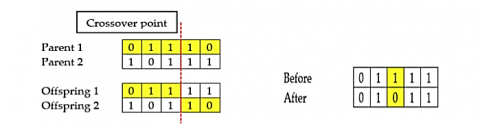Figure 6. Crossover operation in the proposed method 

The algorithm applied in the proposed method is shown in Figure 7.

## 7.png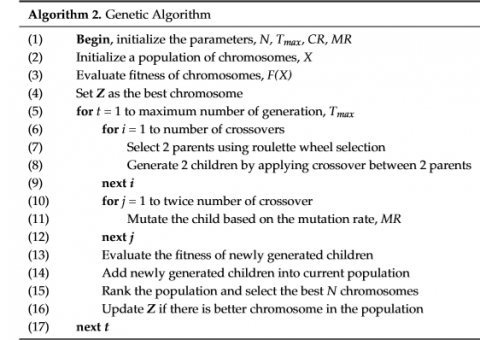Figure 7. Crossover operation in the proposed method 

However, in order to improve the classification accuracy, feature reduction method which was described above and known as the modified genetic algorithm, is presented in the study  and has been applied in the proposed method.

4.5 Features normalization

Given that the large amounts in the feature extraction stage may affect the accuracy of the classification, data normalization is applied:

$\hat{x}=\frac{x_{i}-x_{\min }}{x_{\max }\,\,-x_{\min }}$       (24)

In the above relation, x represents the new value in the attribute. x_i describes the initial value of the property. xmin and xmax are considered the smallest and largest values.

5. Experimental Result

In this section, the results of experiments related to leaf disease of the two datasets obtained from rice and tomato leaves, are tested. Moreover, in order to show the performance comparisonof different classifier and the KNN algorithm, experiments were taken. The parameters that the analysis was done according to them were including the amount of time that a clasifier takes to consture a model, false- positive rate, true positive rate, and accuracy. True Positive (TP) indicates the examples that are correctly predicted as normal. True Negative (TN) express the examples and cases which are correctly predicted as a leaf diseas. False Positive (FP) identifies the instances that fail to be a true prediction. For example, cases that are predicted as diseased, whereas they are not. On the other hand, False Negative (FN) is for the cases in which plants have been predicted to be normal while they are diseased in real. Accuracy, "Specificity" and "Specificity" is used Eqns. (25)-(27).

Accuracy $=\frac{T P+T N}{N}$    (25)

Specificity $=\frac{\mathrm{T}_{P}}{\mathrm{~T}_{\mathrm{P}}+F_{\mathrm{N}}}$         (26)

Specificity $=\frac{\mathrm{T}_{\mathrm{N}}}{\mathrm{T}_{\mathrm{P}}+F_{\mathrm{N}}}$      (27)

5.1 Investigation of the proposed method

In this section, the proposed method is first examined using improved k-means segmentation. Figures 8 shows the input images related to the diseased leafs of tomato and rice plant.

Figure 9 illustrates the process of segmentation by improved k-means on both leaves of tomato and rice, respectively.

As shown in Figure 9, in both types of leaves, the number of clusters is considered to be 3 in which the leaf disease is well segmented, and this can be very effective in the feature extraction process. However, in each channel, we can get comprehensive features. The process of feature selection leads to the selection of the most optimal features, which will be shown later by the feature selection function via GA in Figure 9 over 200 repetitions.

## 8a.png(a) tomato disease leaf

## 8b.png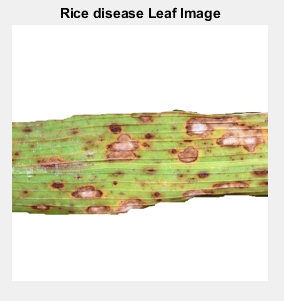(b) rice disease leaf

Figure 8. Input images

## 9a.png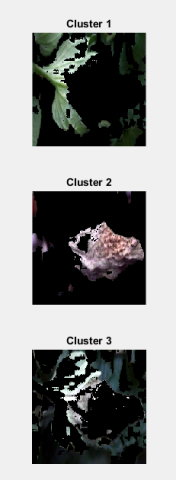(a). tomato segmentation with improved k-means

## 9b.png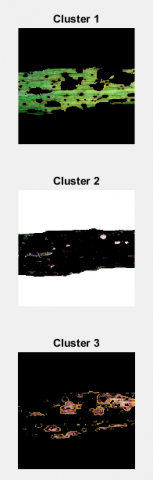(b). rice segmentation with improved k-means

Figure 9. Segmentation images

## 10.pngFigure 10. Fitness plot for the proposed method

As can be seen in Figure 10, the feature selection function in the proposed method has been optimized through genetic algorithm and becomes ideally converged after 200 times of replications. This is while, during repetition process from 120 to 200 the function is completely convergent and shows excellent performance.

5.2 Comparison of the proposed approach with different classifiers

In this section, the performance of three criteria of accuracy, Specificity and sensitivity in the proposed method has been investigated on both tomato and rice leaf disease datasets.

In the proposed method, first, the accuracy obtained from different classifications in Figures 11 and 12 is compared.

## 11.png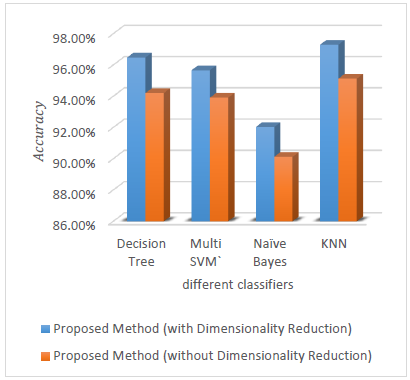Figure 11. Comparison of the accuracy of the proposed method obtained from the different classifiers using Tomato dataset

## 12.png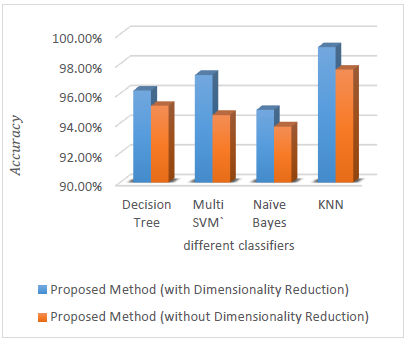Figure 12. Comparison of the accuracy of the proposed method obtained from the different classifiers using Rice dataset

## 13.png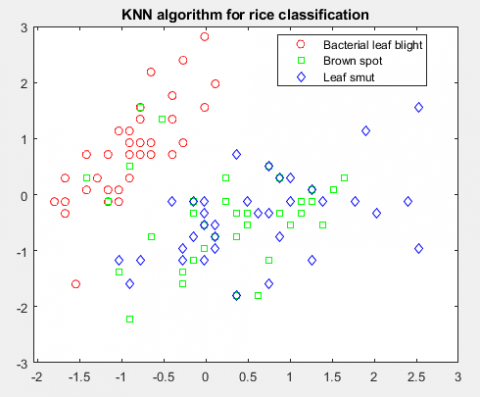Figure 13. Plot scatter for rice classification

As shown in Figures 11 and 12, the KNN classifier has the best performance among the other classifications. Other categories, like the decision tree, are in second rank. In general, the decision tree and KNN classification methods have a very high speed when the data set contains different classes. However, SVM fails to have such feature. Also, among the categories, New Bayesian has the worst performance.

Thus, Figure 13 shows the superiority of the KNN classification function for a set of rice images in three categories of labels.

As can be seen from Figure 13, the KNN classifier has been able to segregate the features of each class well, so each has the same distribution.

Tables 1 and 2 show the details of quantitative criteria for tomato and rice leaf disease data sets.

According to Tables 1 and 2 for the tomato and rice data set, the proposed method has a very good performance in terms of accuracy and detection and sensitivity using the KNN classifier.

Table 1. Comparison of the proposed approach with different classifiers  via Tomato dataset

 Method Tomato Dataset Proposed method (with Dimensionality Reduction) Classifier Accuracy Specificity sensitivity Decision Tree 96.47% 95.15% 98.23% Multi SVM 95.65% 94.52% 95.25% Naïve Bayes 92.02% 93.10% 94.3% KNN 97.29% 96.34% 98.74% Proposed method (without Dimensionality Reduction) Decision Tree 94.20% 93.40% 95.99% Multi SVM 93.90% 94.61% 95.87% Naïve Bayes 90.11% 91.35% 92.67% KNN 95.12% 95.05% 96.27%

Table 2. Comparison of the proposed approach with different classifiers via Rice dataset

 Technique Rice Dataset Proposed method (with Dimensionality Reduction) Classifier Accuracy Specificity sensitivity Decision Tree 96.20% 98.54% 99.30 Multi SVM 97.25% 98.26% 99.12 Naïve Bayes 94.89% 95.23% 96.23 KNN 99.12% 99.47% 99.89 Proposed method (without Dimensionality Reduction) Decision Tree 95.17% 95.41% 95.74 Multi SVM 94.55 % 94.40% 94.78 Naïve Bayes 93.77% 94.38% 94.88 KNN 97.60% 96.47% 97.23

5.3 Comparison of the proposed method with other methods

In this section, the proposed method is compared with other methods. The accuracy of the proposed method using data sets of rice has been compared with CNN methods , Decision Tree-based Machine Learning Algorithms  and improved random forest , Detection and Classiﬁcation of Rice Plant Diseases , Deep Neural Network Algorithm , An Approach Using Textural Features , and tomato data set has been compared with Computer Vision Based Detection and Classification , Automatic Tomato Plant Leaf Disease and pests , Deep Neural Network-Based Tomato Plant Diseases ,Tomato plant disease classification methods in digital images , Automatic Tomato Plant Leaf Disease .

Tables 3 and 4 show a good comparison of the accuracy of different rice and tomato datasets, respectively.

It should be noted that the data sets of the methods compared for both rice and tomato diseases are the same as the proposed method, and therefore the experiments are the same in terms of data sets.

Table 3. Comparison of proposed method with other methods using Rice dataset

 Method Rice Dataset Accuracy  93.3%  76.19%  97.80%  93.33%  96%  94.16% Proposed Method 99.12%

Table 4. Comparison of proposed method with other methods using Tomato dataset

 Method Tomato Dataset Accuracy  97.3%  96.00%  89.00%  79%  87.3% Proposed Method 97.29%

As can be seen in Tables 3 and 4, the proposed method performs better in the rice data set and the method  despite being very close to the proposed method, is weaker in the classification process, because of extracting less features in comparison to the proposed method. In addition, CNN has more time complexity in the classification process. The same is true for the tomato leaf disease data set obtained from Method . Therefore, the proposed method, by extracting appropriate features, has been able to extract 99.12 for rice leaf disease data set and 97.29 for tomato leaf disease data set.

6. Conclusions

In this paper, the image processing method in diagnosing and determining the type of two diseases in datasets of the tomato and rice leaves was evaluated by k-mean segmentation and extraction of comprehensive characteristics. The results showed that the image processing method can distinguish healthy leaves from infected leaves with high accuracy and also diagnose the type of disease with high accuracy. It seems that due to the fact that plant diseases are spread regionally in the field, image processing technique and visual machine technology can be applied for accurately and timely determining these diseases in the field and therefore lead to minimize crop losses and moreover, the need for chemicals could be reduced through Applying regional farm management. In future work, experiments will be tested on other datasets using depth learning classification.

References

 Ramesh, S., Vydeki, D. (2020). Recognition and classification of paddy leaf diseases using Optimized Deep Neural network with Jaya algorithm. Information Processing in Agriculture, 7(2): 249-260. https://doi.org/10.1016/j.inpa.2019.09.002

 Parraga-Alava, J., Cusme, K., Loor, A., Santander, E. (2019). RoCoLe: A robusta coffee leaf images dataset for evaluation of machine learning based methods in plant diseases recognition. Data in Brief, 25: 104414. https://doi.org/10.1016/j.dib.2019.104414

 Saleem, G., Akhtar, M., Ahmed, N., Qureshi, W.S. (2019). Automated analysis of visual leaf shape features for plant classification. Computers and Electronics in Agriculture, 157: 270-280. https://doi.org/10.1016/j.compag.2018.12.038

 Petrellis, N. (2019). Plant disease diagnosis for smart phone applications with extensible set of diseases. Applied Sciences, 9(9): 1-22. https://doi.org/10.3390/app9091952

 Petrellis, N. (2017). A smart phone image processing application for plant disease diagnosis. 2017 6th International Conference on Modern Circuits and Systems Technologies (MOCAST), pp. 1-4. https://doi.org/10.1109/MOCAST.2017.7937683

 Vaishnnave, M.P., Devi, K.S., Srinivasan, P., Jothi, G.A.P. (2019). Detection and classification of groundnut leaf diseases using KNN classifier. 2019 IEEE International Conference on System, Computation, Automation and Networking (ICSCAN), pp. 1-5. https://doi.org/10.1109/ICSCAN.2019.8878733

 Senan, E.M., Al-Adhaileh, M.H., Alsaade, F.W., Aldhyani, T.H.H., Alqarni, A.A., Alsharif, N., Uddin, M.I., Alahmadi, A.H., Jadhav, M., Alzahrani, M.Y. (2021). Diagnosis of chronic kidney disease using effective classification algorithms and recursive feature elimination techniques. Journal of Healthcare Engineering, 2021: 1-10. https://doi.org/10.1155/2021/1004767

 Ramesh, N., Liu, T., Tasdizen, T. (2017). Cell detection using extremal regions in a semisupervised learning framework. Journal of Healthcare Engineering, 2017: 1-13. https://doi.org/10.1155/2017/4080874

 Too J., Abdullah A., Mohd Saad N., Tee W. (2019). EMG Feature Selection and Classification Using a Pbest-Guide Binary Particle Swarm Optimization. Computationpp.1-20. https://doi.org/10.3390/computation7010012

 Zhang, C., Zhang, S., Yang, J., Shi, Y., Jia, C. (2017). Apple leaf disease identification using genetic algorithm and correlation based feature selection method. International Journal of Agricultural and Biological Engineering, 10(2): 75-83. https://doi.org/10.3965/j.ijabe.20171002.2166

 Singh, V. (2019). Sunflower leaf diseases detection using image segmentation based on particle swarm optimization. Artificial Intelligence in Agriculture, 3: 62-68. https://doi.org/10.1016/j.aiia.2019.09.002

 Andrushia, A.D., Patricia, A.T. (2019). Artificial bee colony optimization (ABC) for grape leaves disease detection. Evolving Systems, 11: 105-117. https://doi.org/10.1007/s12530-019-09289-2

 Rafeed Rahma, C., Saha, P., Eunus, M. (2020). Identification and recognition of rice diseases and pests using convolutional neural networks. arXiv:1812.01043v3, Biosystems Engineering.

 Sahith, R., Vijaya, P., Nimmala, S. (2019). Decision tree-based machine learning algorithms to classify rice plant diseases. Journal of Innovative Technology and Exploring Engineering (IJITEE), 9(1): 5365-5368. https://doi.org/10.35940/ijitee.A4753.119119

 Chaudhary, A., Kolhe, S., Kamal, R. (2019). An improved random forest classifier for multi-class classification. Information Processing in Agriculture, 3(4): 215-222. https://doi.org/10.1016/j.inpa.2016.08.002

 Sabrol, H., Satish, K. (2016). Tomato plant disease classification in digital images using classification tree. Conference on Communication and Signal Processing (ICCSP), pp. 1242-1246. https://doi.org/10.1109/ICCSP.2016.7754351

 Fuentes, A., Yoon, S., Lee, Y., Sun Park, D. (2018). High-performance deep neural network-based tomato plant diseases and pests diagnosis system with refinement filter bank. Front. Plant Sci. https://doi.org/10.3389/fpls.2018.01162

 Jia, S., Jia, P., Hu, S., Liu, H. (2019). Automatic detection of tomato diseases and pests based on leaf images. Chinese Automation Congress (CAC), pp. 2537-2510. https://doi.org/10.1109/CAC.2017.8243388

 Prajapati, H.B., Shah, J.P., Dabhi, V.K. (2017). Detection and classification of rice plant diseases. Intelligent Decision Technologies, 11(3): 357-73. https://doi.org/10.3233/IDT-170301

 Fuentes, A., Yoon, S., Cheol Kim, S., Park, D. (2017). A robust deep-learning-based detector for real-time tomato plant diseases and pests recognition. Sensor, https://doi.org/10.3390/s17092022

 Qureshi, M.N., Ahamad, M.V. (2018). An improved method for image segmentation using K-means clustering with neutrosophic logic. Procedia Computer Science, 132: 534-540. https://doi.org/10.1016/j.procs.2018.05.006

 Saleem, G., Akhtar, M., Ahmed, N., Qureshi, W. (2019). Automated analysis of visual leaf shape features for plant classification. Computers and Electronics in Agriculture, 157: 270-280. https://doi.org/10.1016/j.compag.2018.12.038

 Shaikh, J.A., Koleka, U.D. (2017). Palm Print Recognition Using Textural Harlick Feature. International Journal of Applied Engineering Research, 12(11): 2922-2925.

 Bosilj, P., Kijak, E., Lefèvre, S. (2015). Beyond MSER: Maximally stable regions using tree of shapes. Project: Image Indexing and Retrieval with Component Trees. https://doi.org/10.5244/C.29.169

 VijayaLakshmi, B., Mohan, V. (2016). Kernel-based PSO and FRVM: An automatic plant leaf type detection using texture, shape, and color features. Computers and Electronics in Agriculture, 125: 99-112. https://doi.org/10.1016/j.compag.2016.04.033

 Prajapati, H.B., Shah, J.P., Dabhi, V.K. (2017). Detection and classiﬁcation of rice plant diseases. Journal: Intelligent Decision Technologies, 11(3): 357-373. https://doi.org/10.3233/IDT-170301

 Ramesh S., Vydeki, D. (2020). Rice disease detection and classification using deep neural network algorithm. In: Sharma D.K., Balas V.E., Son L.H., Sharma R., Cengiz K. (eds) Micro-Electronics and Telecommunication Engineering. Lecture Notes in Networks and Systems, vol 106. Springer, Singapore. https://doi.org/10.1007/978-981-15-2329-8_56

 Bashir, K., Rehman, M., Bari. M. (2019). Detection and classification of rice diseases: An automated approach using textural features. Mehran University Research Journal of Engineering & Technology, 38(1): 239-250. https://doi.org/10.22581/muet1982.1901.20

 Gayathri Devi, T., Srinivasan, A. (2019). Computer vision based detection and classification of tomato leaf diseases. International Journal of Innovative Technology and Exploring Engineering (IJITEE), 8(11): 3103-3102. https://doi.org/10.35940/ijitee.K2493.0981119

 Jayanthi, M., Shashikumar, D. (2020). Automatic tomato plant leaf disease classification using multi-kernel support vector machine. International Journal of Engineering and Advanced Technology (IJEAT), 9(5): 2249-8958. https://doi.org/10.35940/ijeat.E9689.069520